﻿ 基于改进粒子群算法的风光燃储微电网多目标优化研究 Multi-Objective Optimization of Wind/PV/Gas/Storage Micro-Grid Based on Improved Particle Swarm Optimization

Journal of Electrical Engineering
Vol. 06  No. 04 ( 2018 ), Article ID: 28286 , 9 pages
10.12677/JEE.2018.64045

Multi-Objective Optimization of Wind/PV/Gas/Storage Micro-Grid Based on Improved Particle Swarm Optimization

Hai Xu1, Liang Wang1, Zhen Li2, Shuqing Ding3

1Jiangsu Keneng Power Engineering Consulting Co. LTD., Nanjing Jiangsu

2State Grid Shandong Electric Power Company, Dezhou Power Supply Company, Dezhou Shandong

3Nanjing Special Equipment Safety Supervision and Inspection Institute, Nanjing Jiangsu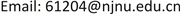Received: Dec. 5th, 2018; accepted: Dec. 20th, 2018; published: Dec. 28th, 2018ABSTRACT

Based on the economy, reliability and environmental protection of independent micro-grid operation, a multi-objective optimal configuration model with solar energy, wind energy, micro gas generator and energy storage as distributed power source was established. By analyzing the operating characteristics of the distributed power supply of wind and light storage, the annual temperature, photovoltaic intensity and wind speed are taken as inputs to obtain the optimal configuration scheme of multi-objective of independent micro-grid. To solve the optimization problem, an improved particle swarm optimization algorithm is proposed. The results show that the improved particle swarm optimization algorithm has the advantages of strong global optimal search ability and fast optimization. The effectiveness of the proposed model and the proposed method is verified by simulation analysis.

Keywords:Multi-Objective Optimization, Wind and Light Storage, Distributed Power Supply, Improved Particle Swarm Optimization

1江苏科能电力工程咨询有限公司，江苏 南京

2国网山东省电力公司德州供电公司，山东 德州

3南京特种设备安全监督检验研究院，江苏 南京1. 引言

2. 风光燃储独立微电网电源模型

2.1. 光伏阵列模型

${P}_{PV}\left(t\right)={\eta }_{PV}{P}_{STC}\frac{{G}_{c}\left(t\right)}{{G}_{STC}}\left[1+k\left({T}_{c}\left(t\right)-{T}_{STC}\right)\right]$ (1)

2.2. 风力发电机模型

${P}_{WG}\left(v\right)=\left\{\begin{array}{l}0,0\le v\left(t\right)\le {v}_{ci},v\left(t\right)\ge {v}_{co}\\ {P}_{r}\frac{v\left(t\right)-{v}_{ci}}{{v}_{r}-{v}_{ci}},{v}_{ci}\le v\left(t\right)\le {v}_{r}\\ {P}_{r},{v}_{r}\le v\left(t\right)\le {v}_{co}\end{array}$ (2)

2.3. 微型燃气轮机模型

${C}_{MT}=\left({C}_{ng}/L\right){P}_{rc}/{\eta }_{e}$ (3)

2.4. 储能装置模型

${P}_{SB}\left(t\right)=\left\{\begin{array}{l}{P}_{SB}\left(t-1\right)+\left({P}_{t}\left(t\right)-\frac{{P}_{L}\left(t\right)}{{\eta }_{inv}}\right)\cdot {\eta }_{sb}\\ {P}_{SB}\left(t-1\right)-\left(\frac{{P}_{L}\left(t\right)}{{\eta }_{inv}}-{P}_{t}\left(t\right)\right)\end{array}$ (4)

3. 微电网的多目标优化配置模型

3.1. 目标函数

$\mathrm{min}F\left(X\right)=\mathrm{min}\underset{i=1}{\overset{3}{\sum }}{w}_{i}{f}_{i}\left(x\right)$ (5)

3.1.1. 等年值设备投资成本

${f}_{1}\left(x\right)={C}_{DG}+{C}_{OM}+{C}_{R}+{C}_{F}$ (6)

3.1.2. 停电损失费用

${f}_{3}\left(x\right)=\underset{i=1}{\overset{8760}{\sum }}{P}_{LOLP}\cdot C$ (7)

3.1.3. 污染排放成本

${f}_{2}\left(x\right)=\underset{i\in {N}_{DG}}{\sum }\underset{j\in m}{\sum }\left({s}_{j}{v}_{j}\right){x}_{i}{p}_{i}$ (8)

3.2. 约束条件

3.2.1. 分布式电源出力约束

${P}_{i}\left(t\right)\le {p}_{i}{x}_{i}$ (9)

3.2.2. 分布式电源数目约束

${N}_{DG,\mathrm{min}}\le {N}_{DG}\le {N}_{DG,\mathrm{max}}$ (10)

3.2.3. 储能装置运行约束

$\left\{\begin{array}{l}SO{C}_{\mathrm{min}}\le SOC\left(t\right)\le SO{C}_{\mathrm{max}}\\ {P}_{ch}\left(t\right)\le 0.2{E}_{Bat}/\Delta t\\ {P}_{dch}\left(t\right)\le 0.2{E}_{Bat}/\Delta t\end{array}$ (11)

4. 算法优化

4.1. 基本粒子群算法

$\left\{\begin{array}{l}{v}_{id}^{\left(k+1\right)}={v}_{id}^{\left(k\right)}+{c}_{1}{r}_{1}\cdot \left({p}_{id}^{\left(k\right)}-{x}_{id}^{\left(k\right)}\right)+{c}_{2}{r}_{2}\cdot \left({p}_{gd}^{\left(k\right)}-{x}_{id}^{\left(k\right)}\right)\\ {x}_{id}^{\left(k+1\right)}={x}_{id}^{\left(k\right)}+{v}_{id}^{\left(k\right)}\end{array}$ (12)

4.2. 改进粒子群算法

$\left\{\begin{array}{l}{v}_{id}^{\left(k+1\right)}={w}^{\left(k\right)}\cdot {v}_{id}^{\left(k\right)}+{c}_{1}{r}_{1}\cdot \left({p}_{id}^{\left(k\right)}-{x}_{id}^{\left(k\right)}\right)+{c}_{2}{r}_{2}\cdot \left({p}_{gd}^{\left(k\right)}-{x}_{id}^{\left(k\right)}\right)\\ {x}_{id}^{\left(k+1\right)}={x}_{id}^{\left(k\right)}+{v}_{id}^{\left(k\right)}\end{array}$ (13)

${w}^{\left(k\right)}={w}_{\mathrm{min}}+\left({w}_{\mathrm{max}}-{w}_{\mathrm{min}}\right)×\mathrm{exp}{\left(-25×\left(\frac{k}{{k}_{\mathrm{max}}}\right)\right)}^{3}$ (14)

1) 初始化一个规模为N维数为D的粒子群，随机初始化种群中各粒子的位置和速度。

2) 计算每个粒子的适应值。

3) 对每个粒子将其适应值和其经历的最好位置pbest的适应值进行比较，若较好，则将其作为当前的个体最好位置。

4) 对每个粒子将其适应值和全局经历的最好位置gbest大的适应值进行比较，若较好，则将其作为当前的全局最好位置。

5) 使用式(13)更新每个粒子的速度和位置。

6) 使用式(14)更新惯性权重。

7) 若满足终止条件，搜索停止，输出结果；否则回到步骤2继续执行。

5. 算例分析

5.1. 算例描述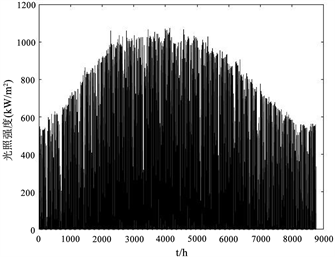Figure 1. Annual light curve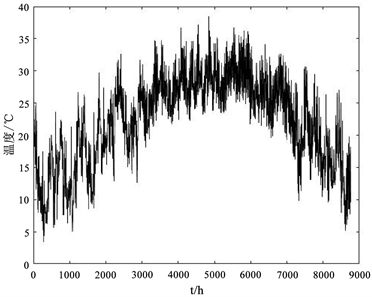Figure 2. Annual temperature gauge curve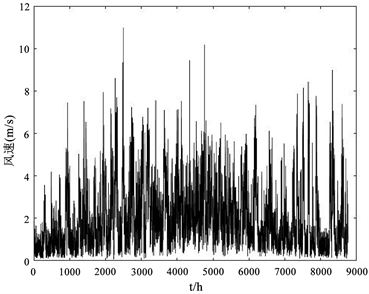Figure 3. Annual wind speed curve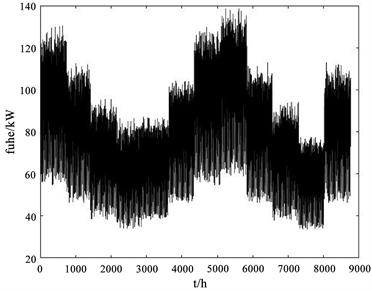Figure 4. Annual electricity load curve

5.2. 优化结果比较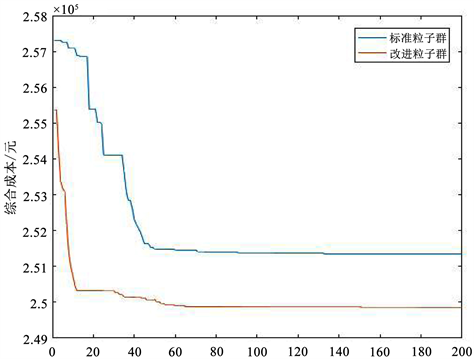Figure 5. Standard particle swarm and improved particle swarm optimization curve

6. 结语

Multi-Objective Optimization of Wind/PV/Gas/Storage Micro-Grid Based on Improved Particle Swarm Optimization[J]. 电气工程, 2018, 06(04): 380-388. https://doi.org/10.12677/JEE.2018.64045

1. 1. 李瑞生, 马凯琪, 于芃, 等. 交直流混合微电网系统建模及协调控制研究[J]. 电测与仪表, 2017, 54(20): 92-99.

2. 2. 吕智林, 谭颖, 李捷, 等. 基于Markov-ELM的独立混合微电网分布式电源多目标容量优化配置[J]. 中国电机工程学报, 2017, 37(7): 1927-1936.

3. 3. 董默. 微电网多目标优化运行研究[J]. 智能电网, 2015, 3(2): 107-111.

4. 4. 张有兵, 包侃侃, 杨晓东, 等. 独立微电网多目标优化配置[J]. 浙江工业大学学报, 2016, 44(6): 619-623.

5. 5. 郭力, 刘文建, 焦冰琦, 等. 独立微网系统的多目标优化规划设计方法[J]. 中国电机工程学报, 2014, 26(6): 85-92.

6. 6. 王成山, 洪博文, 郭力. 不同场景下的光蓄微电网调度策略[J]. 电网技术, 2011, 37(7): 1775-1782.

7. 7. 谭颖, 吕智林, 李捷. 基于改进ELM的风/光/柴/储独立微电网分布式电源多目标容量优化配置[J]. 电力系统保护与控制, 2016, 44(8): 63-70.

8. 8. 宋旭日, 叶林. 风/光/柴多能互补发电系统优化配置研究[J]. 电网与清洁能源, 2011, 27(5): 66-71.

9. 9. Kennedy, J. and Eberhart, R.C. (1995) Particle Swarm Optimization. Proceedings of the International Conference on Neural Networks, Perth, WA, 27 November-1 December 1995, 1942-1948. https://doi.org/10.1109/ICNN.1995.488968

10. 10. 邓辉平, 江冰, 蔡昌春. 海岛智能微电网多源协调优化运行研究[J]. 信息技术, 2017, 15(4): 104-109.

11. 11. Shi, Y. and Eberhart, R.C. (1999) Empirical Study of Particle Swarm Optimization. Proceedings of the 1999 Congress on Evolutionary Computation, Washington, DC, 6-9 July 1999, 1945-1950. https://doi.org/10.1109/CEC.1999.785511